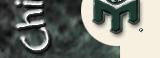# Bayes' Theorem and the Case of the Colored Coins

"You have three coins in a jar: one is red on both sides, one is red on one side and blue on the other, and the last one is blue on both sides. You pull one coin out of the jar at random and slap it onto a table. You see that the top is blue. What is the probability that the bottom is blue also?" Warning: People fight over the solution to this one.

If you take an undergraduate Probability/Statistics course-the real P/S course, in the math department—you will learn Bayes' Theorem in about week four; if you don't get it you'll have trouble passing. Teachers have asked this red-blue question for years, and bright math students get it right. This happens to be more of a probability problem than a statistics problem, but let's not get into that.

Resorting to trials is not necessary. Imagining any situation in which the coin shows red side up is irrelevant. We are being asked to make a probability statement knowing only that a blue side is showing; we are not being asked to consider redoing something that has already occurred. The problem as stated is complete, unambiguous, and bears the writing style of a math teacher. Whether people can accept the correct answer is relevant to the selection of decision makers who process the testimonials of experts. Experts can teach this, but they can't make it "get learned." Let's begin, shall we?

The concept of conditional probability, considered alone, doesn't encompass a general solution as well as does Bayes' Theorem, which extends the concept of conditional probability. We use Bayes' Theorem to make probability statements regarding an observed event that could have been caused in any of several different ways. Given that an event has occurred, we want to know the probability that the cause was "Cause X."

For each cause that we are considering, we need a product: the probability of the cause times the probability of the event given that cause. Notice that we have such a probability product for each cause of interest to us. For our problem, what would these products look like?

A: (probability of drawing a blue/blue coin) times (probability of having some blue side of the blue/blue coin be "up") = 1/3 times 1 = 1/3. Note: The probability of an event that is certain to occur is 1, and the blue/blue coin is certain to come up blue.

B: (probability of drawing a red/blue coin) times (probability of having the blue side of this coin be "up") = 1/3 times 1/2 = 1/6.

C: We ignore the possibility of getting a blue side up from a red/red coin because this cannot happen.

We are now finished with the tricky parts of the problem. Phew! (That wasn't so hard.)

We need to make a fraction to use Bayes' Theorem; the fraction will give a new probability that is the answer to our problem. Put the product for the tested cause ("Is our coin the blue/blue coin?") in the numerator. In the denominator, put the sum of the products for all causes that can produce the "blue side up" event. Do not include causes that cannot produce the event. Figures relating to the red/red coin do not appear in our final fraction.

So our fraction is A/(A + B), or (1/3)/(1/3 + 1/6) = (1/3)/(1/2) = 2/3. Whether you accept this numerical result is of no interest to us, though it pains me to see Mensans tangle over it without the benefit of Mr. Bayes' contribution.

Why don't we like trials? They aren't needed, and in the real world we may not have the luxury of conducting trials. For example, given that a rocket has gone off course and been destroyed, and given the known failure rates of the engine and the navigation hardware, what is the probability that the accident was caused by the engine? by the navigation hardware? Get the right answer or astronauts may die; the rockets are too expensive to allow multiple trials. Furthermore, real-world scenarios may involve dozens of potential failure events and hundreds of possible causes. Don't make a mistake!

By the way, "Bayesian Probability," as it's known, frequently comes up in medicine and scores of other contexts. For example, a smoker's spouse may have been exposed to radiation in the workplace. Given that this nonsmoking spouse has now contracted lung cancer, can we make a reliable statement about which factor was the probable cause? What if the patient is 22 years old? What if the patient is 82? What if two neighbors have the same cancer? What if a sibling does? What if the patient has been taking medicine for a chronic condition? and so on. Bayes' Theorem can help thwart "junk science."

My qualifications for this one are a humble math degree, an exam credit in P/S from the Society of Actuaries, and experience writing proofs on behalf of insurance companies (I no longer do this), but simply knowing the theorem ought to cover it. Using a computer program to simulate this problem is interesting and valid, but it raises a host of other issues regarding computer proofs and it is not needed for this problem, so I won't address it here.

Oh, please don't ask me about Monty Hall's three doors: you can tackle it yourself using Bayes' Theorem.